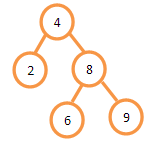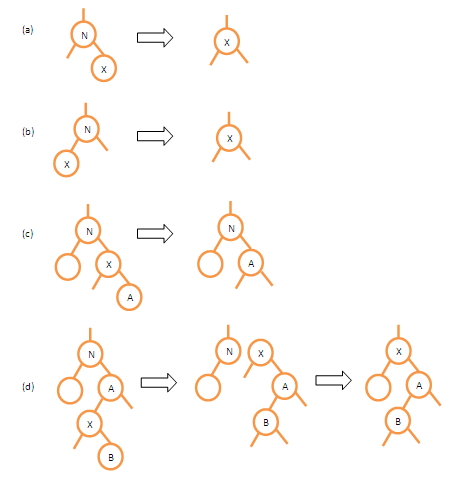# How to implement Binery Search Tree in C# and analysis on running time of basic operations

 Category: Data Structure And Algorithms Tags:Binary Search Tree Code Files

## Introduction

Binary Search Tree is a data structure aims to perform basic operations on tree in time proportional to height of tree. BST supports many operations like search, insert, delete, minimum, maximum. All these operation’s running time is O(log n)  where n is the number of nodes in tree.
In BST a node may have maximum 2 children, left must less or equal to parent and right must grater or equal to parent node this is called BST Property and every BST must satisfy this property. As shown in figure below:## Implementation

In this article we will cover how to implement a BST and almost all operations on a BST. To implement BST we just need a node structure which will create roots and branches so for we will create a class Node:

```/// <summary>
/// Node can be child or root, having a key value,
/// root node's parent will be null
/// </summary>
class Node
{
public int Key;
public Node Parent;
public Node Left;
public Node Right;
}
```

Now we will create a root node where our tree will start getting build in every insertion and deletion, to reach any other node we should have a root node to start, for that we will create a class having root node with all the operation encapsulated, below interface will give you an idea about operations we are going to implement:

```interface IBinerySearchTree
{    Node Root { get; set; }
void Insert(Node node);
void InOrderTreeWalk(Node node);
Node TreeSearch(Node node, int key);
Node MinimumTree(Node node);
Node MaximumTree(Node node);
void TreeDelete(int key);
}
```

Now we will implement these methods in class below:

```class BinerySearchTree : IBinerySearchTree
{
//Root of tree
public Node Root { get; set; }

/// <summary>
/// Constructor
/// </summary>
public BinerySearchTree()
{ }

public BinerySearchTree(int rootKey)
{
Root = new Node() { Key = rootKey };
}
}```

Above class is having constructor where you can initialize root if you want, now we will implement first method Insert of interface IBinerySearchTree inside class BinerySearchTree below:

```/// <summary>
/// Inserts a node at it's correct position
/// </summary>
/// <param name="node">Node which has to be inserted</param>
public void Insert(Node node)
{
Node temp = null;
Node root = Root;

//finding end of the tree to insert
while (root != null)
{
temp = root;
if (node.Key < root.Key)    //According to BST Property
root = root.Left;
else
root = root.Right;
}

/*temp is holding refrence of node at end so
making temp as parent of node which we want to insert*/
node.Parent = temp;

if (temp == null)               //Again According to BST Property
Root = node;
else if (node.Key < temp.Key)
temp.Left = node;
else
temp.Right = node;
}
```

In above method we have to pass a node which has to be inserted, for that we start with root and find an end satisfying BST Properties once we are at end we add this node as left/right child depends on BST Property satisfaction. Now we will implement InOrderTreeWalk :

```/// <summary>
/// Inorder tree walk which prints elements in sorted order
/// </summary>
/// <param name="node">Node where to start walk</param>
public void InOrderTreeWalk(Node node)
{
if (node != null)
{
InOrderTreeWalk(node.Left);     //Recursive
Console.WriteLine(node.Key);   //Print
InOrderTreeWalk(node.Right);    //Recursive
}
}
```

Above method is just 3 line of code 1st and 3rd line inside if condition is recursive and 2nd line is actually sandwiched that is printing key value of current node. This gives us a sorted output, Now we will implement TreeSearch :

```/// <summary>
/// Search node using a key, returns a match
/// </summary>
/// <param name="node">Node where to start search</param>
/// <param name="key">Search key</param>
/// <returns>Match</returns>
public Node TreeSearch(Node node, int key)
{
if (node == null || node.Key == key)
return node;
else if (node.Key < key)
return TreeSearch(node.Right, key);
else
return TreeSearch(node.Left, key);
}
```

Searching a node is very easy and can be completed in O(log n) time. It follows BST Property, if key is greater than current node’s key obviously it will search in right subtree else in left subtree and so on until an exact match is encountered. Next two methods MinimumTree and MaximumTree are implemented below:

```/// <summary>
/// Returns minimum key value node
/// </summary>
/// <param name="node">Node where to start search</param>
/// <returns>Match</returns>
public Node MinimumTree(Node node)
{
if (node.Left != null)
return MinimumTree(node.Left);        //Minimum will be leftmost child
return node;
}

/// <summary>
/// Returns maximum key value node
/// </summary>
/// <param name="node">Node where to start search</param>
/// <returns>Match</returns>
public Node MaximumTree(Node node)
{
if (node.Right != null)
return MaximumTree(node.Right);     //Maximum will be rightmost child
return node;
}
```

It is obvious leftmost child will be smallest and rightmost child will be largest which is implemented in above methods, now we will implement TreeDelete which deletes a node from tree, for that first we have to implement a method called Transplant :

```/// <summary>
/// Transplant a node with another
/// </summary>
/// <param name="u">node which has to transplant</param>
/// <param name="v">node which has to be placed in place of other</param>
private void Transplant(Node u, Node v)
{
if (u.Parent == null)   //If u is root itself
Root = v;
else if (u == u.Parent.Left)    //If u is left child of it's parent
u.Parent.Left = v;          //Making v as left child of u's parent(replacing u)
else
u.Parent.Right = v;

if (v != null)
v.Parent = u.Parent;
}
```

Actually we might have three scenarios when we go for deletion(below we are referring term node is a node we have to delete):

1. If node is having one of Left/Right Child then we directly remove the node and place It's Left/Right child (whichever is available) at node's position.
2. If node is having both Left and Right children then we will look for it's successor in node's right subtree, once we have successor:
• If successor is right child of node(node we want to delete) then we will just transplant node with successor and make node's left subtree as successor's left subtree
• If successor is in node's right subtree but not right child of node then first we replace successor with its own right child then we replace successor with node which we have to delete

In below figure we can see all the scenarios where we are trying to delete node N and X is the successor.Transplant is a private method which is used by TreeDelete method, Transplant actually does place node v in place of node u. This method first checks if parent of u is null means it is root node then sets root to node v. If u is left node of it’s parent then it makes v as left child for u’s parent and same if u is right child then v will be new right child in place of u. Now we will implement TreeDelete :

```/// <summary>
/// Deletes a key matching node
/// </summary>
/// <param name="key">key to delete</param>
public void TreeDelete(int key)
{
Node nodeToDelete = TreeSearch(Root, key);          //Searching which node to be deleted
if (nodeToDelete.Left == null)                      //If Left child is null
Transplant(nodeToDelete, nodeToDelete.Right);   //Then transplant its right child on it's position
else if (nodeToDelete.Right == null)
Transplant(nodeToDelete, nodeToDelete.Left);
else                                                //If both children are available
{
Node min = MinimumTree(nodeToDelete.Right);     //Find minimum node in right subtree(successor)

if (min.Parent != nodeToDelete)                 //if minimum is not a child of node we want to delete
{
Transplant(min, min.Right);                 //Making min's right at min's place
min.Right = nodeToDelete.Right;
min.Right.Parent = min;
}

Transplant(nodeToDelete, min);          // If minimum is a child of node we want to delete then it will directly transplant
min.Left = nodeToDelete.Left;           //Making min's left is node's left which we want to delete
min.Left.Parent = min;
}
}
```

TreeDelete is deleting the node as per three scenarios described above. If and Else If conditions are implemented as shown in fig a and b, Else condition is performing delete as per fig c and d where we have to find successor in right subtree and delete nodes accordingly. Let's run above code:

`IBinerySearchTree bst = new BinerySearchTree();bst.Insert(new Node() { Key = 20 });bst.Insert(new Node() { Key = 15 });bst.Insert(new Node() { Key = 25 });bst.Insert(new Node() { Key = 10 });bst.Insert(new Node() { Key = 30 });bst.Insert(new Node() { Key = 5 });bst.Insert(new Node() { Key = 35 });bst.InOrderTreeWalk(bst.Root);Console.ReadLine();`

Output

5

10

15

20

25

30

35

Always remember in-order walk of binary tree can be used to sort the elements so you can see in above output.

## Analysis

As we can see clearly almost all the operations can be done in O(log n) time, Insertion has a loop which takes log n time to find exact place to insert. Same for search, finding maximum and minimum key takes O(log n) time. But InOrderWalk takes O(n) time because it goes through every node and prints key value.

 Like0 PeopleNikhil Joshi Ceo & Founder at Dotnetlovers Atricles: 146 Questions: 16 Given Best Solutions: 16 *

Login via:x